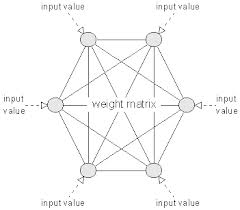## Monday, March 24, 2014

### The Do-Anything-Machine and the Hopfield Neural Network

Machine learning has become a new fascination for me in the past few months.  I have delved into the complicated world of solving problems with neural nets, genetic algorithms and clustering techniques.  Recently, I started work on the Do-Anything-Machine, a project started by Eric Neuman to build an artificial intelligence for solving any type of problem.  This is clearly a huge task, so it has been open-sourced.  To get involved, I have developed this method of solving the N-Queens problem.

The problem states that there are a number of ways to place N number of Queens onto a chess board with NxN squares, such that none of the queens can capture each other.  One way of solving this problem is by using a Hopfield Neural Network configuration.  A Hopfield net is a single-layer network in which all of the neurons are connected.  In our case, each neuron represents a square on the board, so there are N x N neurons.  Each neuron connection is weighted in a way that all of diagonal, horizontal and vertical squares from the initial square are added into that neuron, and the rest are weighted with zero.   The inputs are a random binary array, where each value in the array is an input into one of the neurons.  The output is also a binary array, with each value being an output from one of the neurons.This is an example of a Hopfield network with 6 neurons. (http://www.nnwj.de/hopfield-net.html)
The general method has two parts:
- The Internal process
- The External process

The internal process is an asynchronous method of changing the output of each neuron.
1. A random neuron is picked
2. The input potential of the neuron is calculated by summing the values of all of the horizontal, vertical, and diagonal queens from that square
3. This gives a value that is fed through a sigmoid function
4. The resulting output is then fed back as an input
5. The process repeats

The External process is performed after N x N iterations of the internal process.
1. The internal process is performed N x N times so that the inputs have a chance to evenly distribute
2. The energy (or cost function) of the entire board is calculated
3. If after 3 External iterations, the change in energy is zero, then the system has stabilized, and you have reached local minima
4. If it has not stabilized, then repeat the external process

Due to the amount of randomness, the system converges in anywhere from 3-25 external iterations.  The code for the initial implementation is here.  Please check it out and join in on the project!